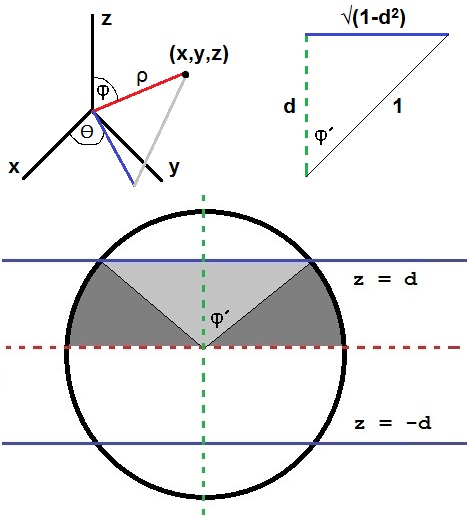## Sunday, February 10, 2013

### Solution to Sphere Problem (Problem # 433)

Here is a statement of the problem:

A sphere of radius 1 is cut by two parallel planes into three parts. The volume of the part between the two planes is exactly half the volume of the whole sphere. What is the smallest possible distance between the two planes?

I attempt to use calculus to solve this problem. I make the approach of setting up a triple integral in spherical coordinates to find the volume of the region sandwiched between the two planes and the unit sphere in order to find the distance between the two planes.

Recall that in spherical coordinates a point, (x,y,z),  in R^3 is represented by the parameters (ρ,φ,Ө). ρ is just the distance between the origin and (x,y,z), φ the angle the red line makes with the positive z-axis (see figure below), and Ө the angle the blue line makes with the positive x-axis in the x-y plane. In spherical coordinates the equation of a plane must be written using the three parameters (ρ,φ,Ө). For example, the plane z = h (where h is any nonzero real number) is defined by ρ*cos φ = h. In terms of ρ, the equation of the plane in spherical coordinates is ρ = h/cos φ. This will prove helpful when I set up the first triple integral. I explain my approach below.1.2.3.